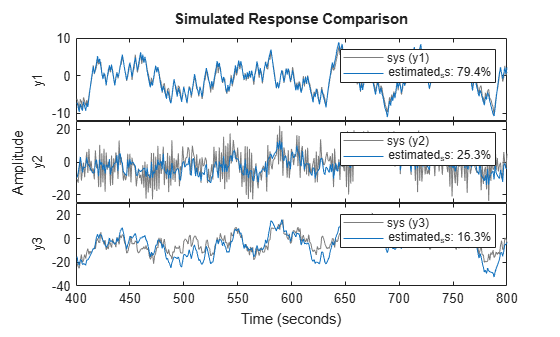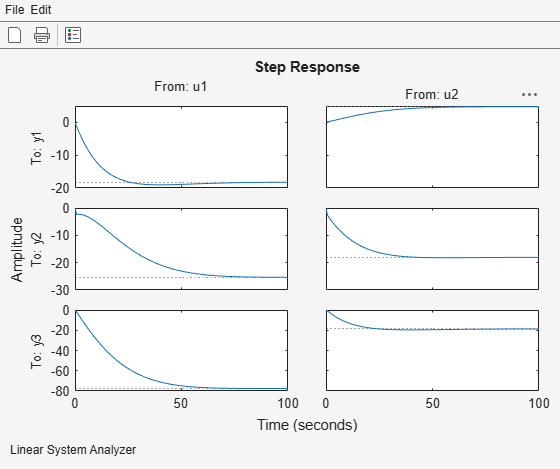## Create and Plot Identified Models Using Control System Toolbox Software

This example shows how to create and plot models using the System Identification Toolbox software and Control System Toolbox software. The example requires a Control System Toolbox license.

Construct a random numeric model using the Control System Toolbox software.

```rng('default'); sys0 = drss(3,3,2);```

`rng('default')` specifies the setting of the random number generator as its default setting.

`sys0` is a third-order numeric state-space model with three outputs and two inputs.

Convert `sys0` to an identified state-space model and set its output noise variance.

```sys = idss(sys0); sys.NoiseVariance = 0.1*eye(3);```

Generate input data for simulating the output.

`u = iddata([],idinput([800 2],'rbs'));`

Simulate the model output with added noise.

```opt = simOptions('AddNoise',true); y = sim(sys,u,opt);```

`opt` is an option set specifying simulation options. `y` is the simulated output for `sys0`.

Create an input-output ( `iddata` ) object.

`data = [y u];`

Estimate the state-space model from the generated data using `ssest` .

`estimated_ss = ssest(data(1:400));`

`estimated_ss` is an identified state-space model.

Convert the identified state-space model to a numeric transfer function.

`sys_tf = tf(estimated_ss);`

Plot the model output for identified state-space model.

`compare(data(401:800),estimated_ss)`Plot the response of identified model using the Linear System Analyzer.

`linearSystemAnalyzer(estimated_ss);`## SupportGet trial now